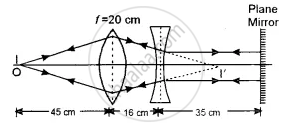# The Figure Below Shows the Positions of a Point Object O, Two Lenses, a Plane Mirror and the Final Image I Which Coincides with the Object. the Focal Length of the Convex Lens is 20 Cm. - Physics (Theory)

Numerical

The figure below shows the positions of a point object O, two lenses, a plane mirror and the final image I which coincides with the object. The focal length of the convex lens is 20 cm. Calculate the focal length of the concave lens.#### Solution

Since the image of the object coincides with the object itself, the rays of light passing through the lens combination strike the mirror normally. The point I’ where the rays of light are converged by the convex lens must be the focus of the concave lens because the emergent rays are parallel to the principal axis. For the convex lens.

u = - 45 cm, f = 20 cm, v = ?

Now, 1/v - 1/u = 1/f

1/v + 1/45 = 1/20

1/v = 1/20 - 1/45 = ( 9 - 4 )/180 = 5/180

∴ v = 180/5 = 36 cm

Now, distance between the two lenses is 16 cm.

∴ Focal length of the concave lens = 36 – 16 = 20 cm

Since the lens is concave, f = – 20 cm

Concept: Ray Optics - Mirror Formula
Is there an error in this question or solution?